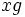# Tour:Left coset of a subgroup

(diff) ← Older revision | Latest revision (diff) | Newer revision → (diff)
We now proceed to a very important notion: the notion of left coset of a subgroup. Given below ar esome equivalent definitions of left coset. There's also a link to a page explaining why the definitions are equivalent. Read the definitions below carefully. There are also some other important facts stated about left cosets, that we'll be encountering again soon. Go through them and mull them in the mind. Proceed to Guided tour for beginners:Left cosets are in bijection via left multiplication OR return to Guided tour for beginners:Union of two subgroups is not a subgroup

## Definition

### Definition with symbols

Let$H$ be a subgroup of a group$G$. Then, a left coset of$H$ is a nonempty set$S$ satisfying the following equivalent properties:

•$x^{-1}y$ is in$H$ for any$x$ and$y$ in$S$, and for any fixed$x \in S$, the map$y \mapsto x^{-1}y$ is a surjection from$S$ to$H$
• There exists an$x$ in$G$ such that$S = xH$ (here$xH$ is the set of all$xh$ with$h \in H$)
• For any$x$ in$S$,$S = xH$

### Equivalence of definitions

For full proof, refer: Equivalence of definitions of left coset

## Facts

### Left congruence

The left cosets of a subgroup are pairwise disjoint, and hence form a partition of the group. The relation of being in the same left coset is an equivalence relation on the group, and this equivalence relation is termed the left congruence induced by the subgroup.

### Relation with right coset

Every subset that occurs as a left coset of a subgroup also occurs as a right coset. In fact, the left coset$xH$ occurs as the right coset$(xHx^{-1})x$ with$xHx^{-1}$ being the new subgroup.

## Numerical facts

### Size of each left coset

Let$H$ be a subgroup of$G$ and$x$ be any element of$G$. Then, the map sending$g$ in$H$ to$xg$ is a bijection from$H$ to$xH$.

For full proof, refer: Left cosets are in bijection via left multiplication

### Number of left cosets

The number of left cosets of a subgroup is termed the index of that subgroup.

Since all left cosets have the same size as the subgroup, we have a formula for the index of the subgroup when the whole group is finite: it is the ratio of the order of the group to the order of the subgroup.

This incidentally also proves Lagrange's theorem -- the order of any subgroup of a finite group divides the order of the whole group.

## Natural isomorphism of left cosets with right cosets

There is a natural bijection between the set of left cosets of a subgroup and the set of right cosets of that subgroup. This bijection arises from the natural antiautomorphism of a group defined by the map sending each element to its inverse. Further information: Left and right coset spaces are naturally isomorphic